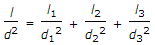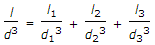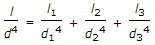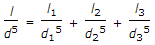# Mechanical Engineering - Hydraulics and Fluid Mechanics - Discussion

1.

A compound pipe of diameter d1, d2 and d3 having lengths l1, l2 and l3 is to be replaced by an equivalent pipe of uniform diameter d and of the same length (l) as that of the compound pipe. The size of the equivalent pipe is given by

 [A].[B].[C].[D].Answer: Option D

Explanation:

No answer description available for this question.

 Gowtham said: (Oct 6, 2013) The discharge will be same and by the equation q=4flv^2/d^5 we get answer.

 Poonam said: (Jan 8, 2014) If there have many pipes. Than what will be answer?

 Pranav said: (Oct 5, 2015) For '1, 2, 3,....N' pipes, having 'L1, L2, L3,....Ln' lengths & 'd1, d2, d3,....dn' dia. In order to replaced by single pipe of L & D length & dia resp. L/D^5 = (l1/d1^5)+(l2/d2^5)+(l3/d3^5)+.....(ln/dn^5).

 Hari said: (Apr 17, 2018) Fluid entering is equal to fluid exit, i.e discharge remains constant, so apply the equation of discharge and arrange it.

 Karthick said: (Sep 27, 2018) Is above formulae used for pipes in parallel as well?

 Taher said: (Jun 28, 2020) Above equation is for parallel pipes.

#### Post your comments here:

Name *:

Email   : (optional)

» Your comments will be displayed only after manual approval.

#### Current Affairs 2021

Interview Questions and Answers